### IMO Shortlist 2018 problem N4

Kvaliteta:
Avg: 0,0
Težina:
Avg: 7,0

Let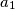$a_1$,$a_2$,$\ldots$ be an infinite sequence of positive integers. Suppose that there is an integer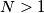$N > 1$ such that, for each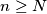$n \geq N$, the number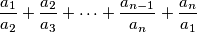is an integer. Prove that there is a positive integer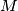$M$ such that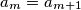$a_m = a_{m+1}$ for all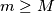$m \geq M$.

Izvor: https://www.imo-official.org/problems/IMO2018SL.pdf